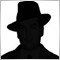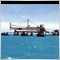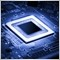• Visão global
• Comentários (6)
• Discussão (11)

# SinFractal7TF

The indicator differs from the classic Fractals indicator by the availability of displaying from one to seven fractal curves (SinFractal) in the additional chart window, which are based on 7 different timeframes and their resulting curve(SinFractal R). Thanks to the applied sine transforms, the values of the curves located within a strictly limited range: from -100 to +100. Settings allow to disable the curves which are not necessary for the trader's strategies. For ease of perception, the additional window of the indicator does not display the curves from the timeframes lower than the chart timeframe the indicator is running on. It is possible to display a curve from a single timeframe. In this case, the curve will be the resulting one. Also, the additional window displays three levels, specified in the settings, alerts and sound notifications may be generated when the resulting curve crosses them. The settings allow to both smooth the displayed curves using ЕМА, and to change their "sensitivity". Depending on the settings, the indicator is able to generate signals formed in various ways: by the curves crossing the specified levels, by the convergence and divergence of the curves of different timeframes, by the change in the location of the different curves relative to the resulting curve, by the intersections of different curves, divergences, etc. Observe, try and choose the signals that are the clearest and closest to your strategies.

The SinFractal curves are calculated by the formula:

SinFractal = 100*Sin(a) = 100*b/с,

where:

с - segment drawn from the candlestick closing price, where the last fractal appeared, to the closing price of the next calculated candlestick;

b - segment equal to the difference between the closing price of the last fractal and the closing price of the next candlestick, which appears after that last closed fractal;

h - segment equal to the number of bars from the last fractal to the next calculated candlestick;

a - angle between the segment с and the segment h.

The resulting curve SinFractal R, calculated by the formula:

SinFractal R = (SinFractal TF1 + SinFractal TF2 + SinFractal TF3 + SinFractal TF4 + SinFractal TF5 + SinFractal TF6 + SinFractal TF7) / 7

where SinFractal TF is the curve calculated for the specified timeframe.

### The indicator parameters

• ЕМА period - period of the ЕМА used for smoothing. Recommended values – from 1 to 20;
• Sensitivity Coefficient – sensitivity coefficient. Recommended values – from 1 to 12;
• TF_0_ - TF_6_ - parameters with a drop-down menu to select the timeframes. When the "qurent" value is set, the selected timeframe is not used (except for the very first, which is used permanently);
• Maximum level - level of the maximum value of the indicator curve, exceeding which generates an Alert. Recommended values – from +30 to +100.
• Minimum level – level of the minimum value of the indicator curve, exceeding which generates an Alert. Recommended values – from -30 to -100.
• Alert zero level – enable/disable Alert that notifies about the intersection of the zero level by the indicator curve.
• Alert max level – enable/disable Alert that notifies about the intersection of the maximum value level by the indicator curve.
• Alert min level – enable/disable Alert that notifies about the intersection of the minimum value level by the indicator curve.
• Maximal bars (0 -all bars) – the number of bars used for the indicator calculation. При значении 0 — the calculation is performed by all bars of the chart.16168
Nikolay Gaylis 2019.01.14 07:05

Usuário não fez qualquer comentário quanto a classificação60
2017.12.28 09:44

Good indicator.12010
2017.12.17 20:21

Usuário não fez qualquer comentário quanto a classificação5675
2017.11.09 13:43

Usuário não fez qualquer comentário quanto a classificação6155
2016.03.27 04:01

Usuário não fez qualquer comentário quanto a classificação7171
2016.03.11 03:40

Usuário não fez qualquer comentário quanto a classificação